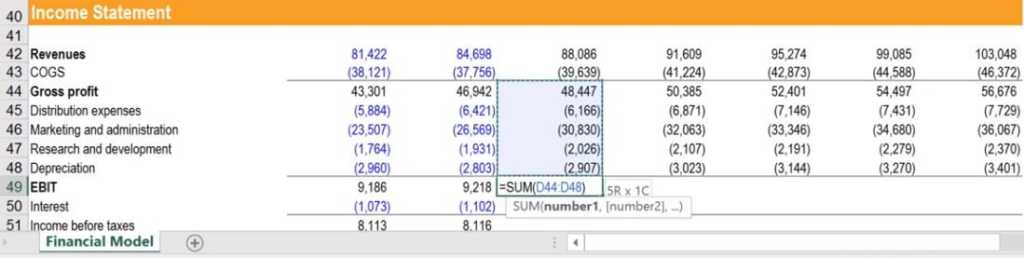# Financial Forecasting

Preparing a prediction of the future

## What Is Financial Forecasting?

Financial forecasting is the processing, estimating, or predicting how a business will perform in the future. The most common type of financial forecast is an income statement, however, in a complete financial model, all three statements are forecasted. In this guide on how to build a financial forecast, we will complete the income statement model from revenue to operating profit or EBIT.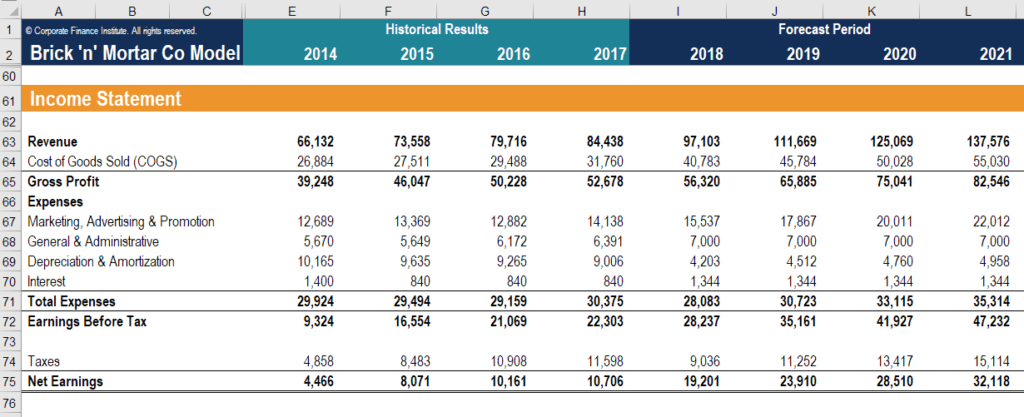### Forecasting Revenue

There are inherent tensions in model building between making your model realistic and keeping it simple and robust. The first-principles approach identifies various methods to model revenues with high degrees of detail and precision. For instance, when forecasting revenue for the retail industry, we will forecast the expansion rate and derive income per square meter.

When forecasting revenue for the telecommunications industry, we will predict the market size and use current market share and competitor analysis. When forecasting revenue for any service industries, we will estimate the headcount and use the income for employee trends.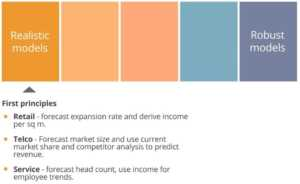On the other hand, the quick and dirty approach to robust models outlines how you can model revenues in a much more straightforward way with the benefit that the model will be more simple and easy to use. In this approach, users predict future growth based on historical figures and trends.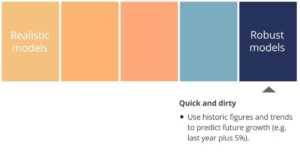### Forecasting Gross Margin and SG&A Expenses

Once we finish forecasting revenues, we next want to forecast gross margins. Gross margins are usually forecast as a percent of revenues. Again, we can use historical figures or trends to forecast future gross margins; however, it is advised to take a more detailed approach, considering factors such as the cost of input, economies of scale, and learning curve. This second approach will allow your model to be more realistic, but also harder to follow.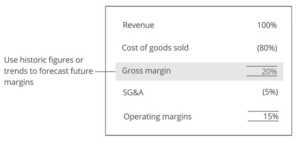The next step is to forecast overhead costs: SG&A expenses. Forecasting Selling, General, and Administrative costs are often done as a percentage of revenues. Although these costs are fixed in the short term, they become increasingly variable in the long term. Therefore, when forecasting over shorter periods (weeks and months), using revenues to predict SG&A may be inappropriate. Some models rather forecast gross and operating margins to leave SG&A as the balancing figure.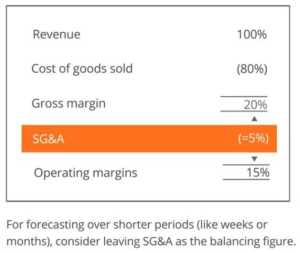### Financial Forecasting Example

Let’s go through an example of financial forecasting together and build the income statement forecast model in Excel. First off, you can see that all the forecast inputs are grouped in the same section called “Assumptions and Drivers.”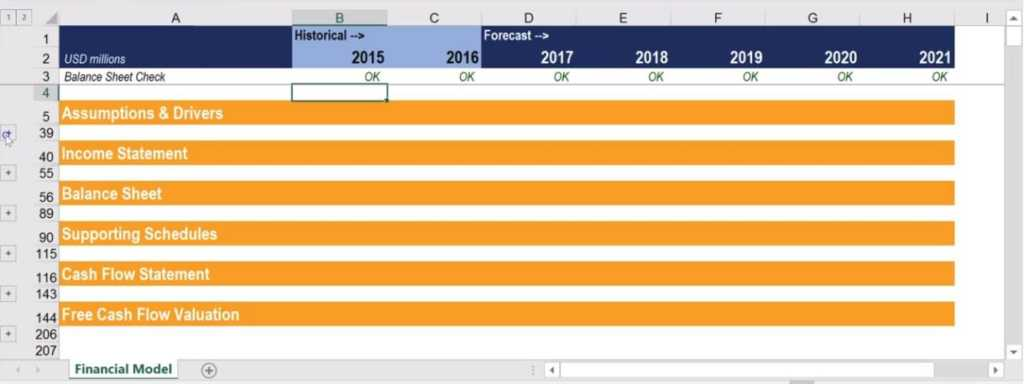I created separate output section groups for the income statement, balance sheet, and cash flow statement. I also created a “Supporting Schedules” section, where detailed processing calculations for PP&E and equity are broken down in order to make the model easier to follow and audit. In this article, we will only work on the assumptions and income statement.

All income statement input assumptions from revenues down to EBIT can be found in rows 8-14. All expenses are being forecasted as a percentage of sales. Only the sales forecast is based on growth over the previous year. My inputs are also ordered in the order they appear on the income statement.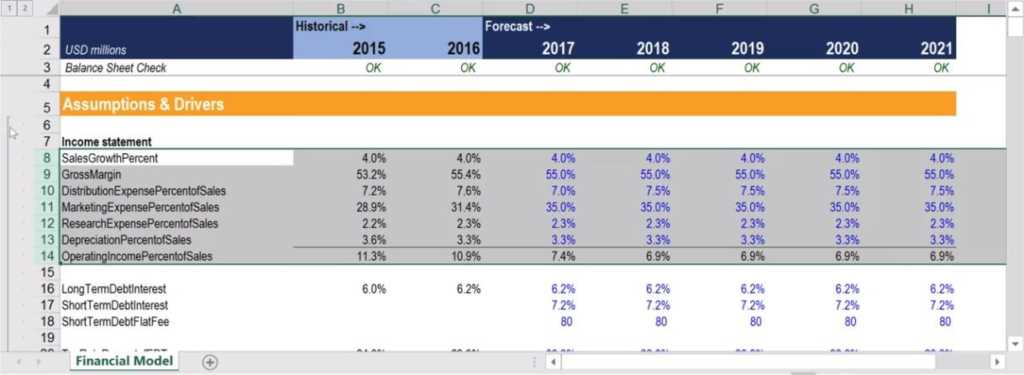Now, let’s move to the “Income Statement” section, where we are going to work on Column D and move downwards. To forecast sales for the first forecast year (in this case 2017), I take the previous year (C42) and grow it by the sales growth assumption in the “Assumptions & Drivers” section. “SalesGrowthPercent” assumption is located in the cell “D8”. Therefore, the formula for the 2017 forecasted revenue is =C42*(1+D8).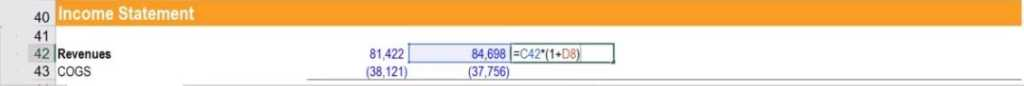I then calculated our Cost of Goods Sold. To calculate the first forecast year’s COGS, we put a minus sign in front of our forecast sales, then multiply by one minus the “GrossMargin” assumption located in cell D9. The formula will read =-D42*(1-D9).

I then sum forecasted sales and COGS to calculate “Gross Profit” located in the cell D44. The formula will read =SUM(D42:D43). A handy shortcut for summing is ALT + =.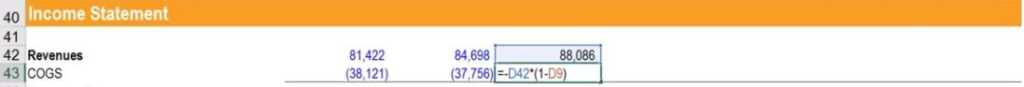Next, I will forecast all the expenses in rows 45 to 48, as a percentage of sales. Let’s first start with “Distribution Expenses”, then copy the formula down to “Depreciation.” To calculate, we subtract the forecast sales and multiply by the appropriate assumption, which in this case is Distribution Expense as a Percent of Sales. The formula will read
=D\$42*D10. Be mindful of the \$ sign because we want to make row 42 of cell D42 an absolute reference. I then copy this formula down, using the shortcut CTRL + D, or fill down.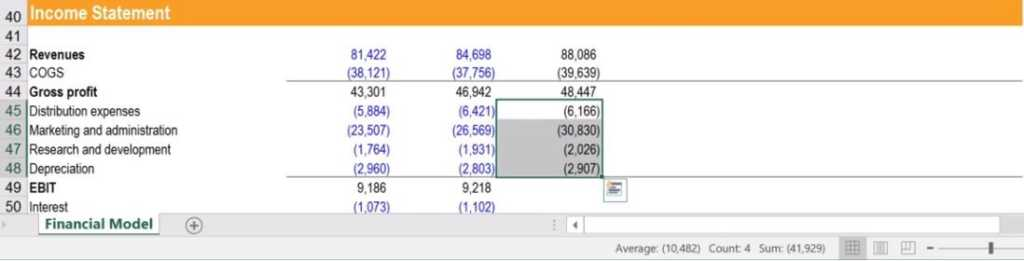Then, over to the right, using the shortcut CTRL + R, or fill right.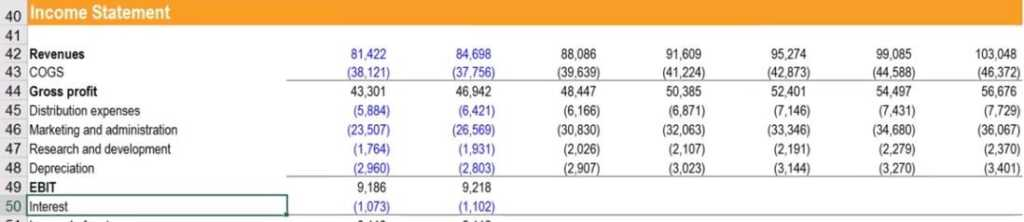Finally, I net gross profit off with all the other operating expenses to calculate EBIT, using =SUM(D44:D48).